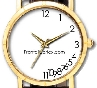Have you seen the parietal watch?

## EEG Frequency Band Definitions

### Topic: Pharmacology

Created on Wednesday, January 3 2007 by jdmiles

An EEG waveform with a frequency of 56 Hz would fit into which frequency band?

A) Gamma

B) Alpha

C) Beta

D) Delta

E) Theta

This question was created on January 03, 2007 by jdmiles.

### A) gamma This answer is correct.

While sources differ on the exact cutoff frequencies, the gamma band is often defined as 40 to 100 Hz.  (See References)### B) alpha This answer is incorrect.

56 Hz is in the gamma band. While sources differ on the exact cutoff frequencies, the gamma band is often defined as 40 to 100 Hz.  (See References)### C) beta This answer is incorrect.

56 Hz is in the gamma band. While sources differ on the exact cutoff frequencies, the gamma band is often defined as 40 to 100 Hz.  (See References)### D) delta This answer is incorrect.

56 Hz is in the gamma band. While sources differ on the exact cutoff frequencies, the gamma band is often defined as 40 to 100 Hz.  (See References)### E) theta This answer is incorrect.

56 Hz is in the gamma band. While sources differ on the exact cutoff frequencies, the gamma band is often defined as 40 to 100 Hz.  (See References)1. Fisch, B. (1999). Fisch and Spehlmann's EEG Primer. 3rd revised and enlarged edition. Elsevier, Amsterdam. (ISBN:0444821481) Advertising: 2. Levin, K.H., and Luders, H.O. (2000). Comprehensive Clinical Neurophysiology. W.B. Saunders, Philadelphia. (ISBN:0721676561) Advertising: 3. Urrestarazu E., Jirsch, J.D., LeVan, P., Hall, J. (2006). High-Frequency Intracerebral EEG Activity (100-500 Hz) Following Interictal Spikes. Epilepsia; 47(9), Pp. 1465�1476. (PMID:16981862)